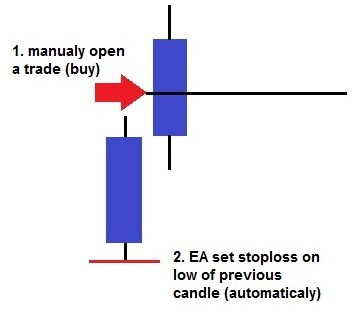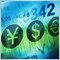# Stoploss EA30

Hi Guys,

i tried to code a stoploss EA, which sets the S/L on the low/high of the previous candle (see pic below).So now I wrote down some code and it does sometimes work. But actually not at every candle.

```//+------------------------------------------------------------------+
//|                                                  SLM.mq4 |
//|                                                                  |
//|                                                                  |
//+------------------------------------------------------------------+
int         Faktor, Digt, cnt;
double         TPp, SLp, Total, i;

//+------------------------------------------------------------------+
//| expert initialization function                                   |
//+------------------------------------------------------------------+
int init(){}

//+------------------------------------------------------------------+
//| expert deinitialization function                                 |
//+------------------------------------------------------------------+
int deinit(){}

//+------------------------------------------------------------------+
//| expert start function                                            |
//+------------------------------------------------------------------+

int start()
{
Comment("Start");

Total=OrdersTotal();
if(Total>0)
{
for(i=Total-1; i>=0; i--)
{
{
if(OrderSymbol() == Symbol()){
if(OrderMagicNumber() != 456976 || OrderMagicNumber() != 659970 || OrderMagicNumber() != 659968 || OrderMagicNumber() != 659969 || OrderMagicNumber() != 4698523){
//    if(OrderComment() == "b"){
{SLp = iLow(NULL, 0, 1);
OrderModify(OrderTicket(),OrderOpenPrice(),NormalizeDouble(SLp,Digits),TPp,0,Blue);
Comment("\nStop Loss will move to BE at ", Low);
}
if(OrderType()==OP_SELL)
{SLp = iHigh(NULL, 0, 1);
OrderModify(OrderTicket(),OrderOpenPrice(),NormalizeDouble(SLp,Digits),TPp,0,Red);
Comment("\nStop Loss will move to BE at ", High);
}
//}
}
}
}
}
}
return(0);
}
//+------------------------------------------------------------------+```391

I don't actually see initialization of TPp variable in your code. Change it to the following and try again. You will need to initialize TPp one If you put it ouside the if blocks.

```
{SLp = iLow(NULL, 0, 1);
TPp = OrderTakeProfit(); //<-----HERE
OrderModify(OrderTicket(),OrderOpenPrice(),NormalizeDouble(SLp,Digits),TPp,0,Blue);
Comment("\nStop Loss will move to BE at ", Low);
}
if(OrderType()==OP_SELL)
{SLp = iHigh(NULL, 0, 1);
TPp = OrderTakeProfit(); //<-----HERE
OrderModify(OrderTicket(),OrderOpenPrice(),NormalizeDouble(SLp,Digits),TPp,0,Red);
Comment("\nStop Loss will move to BE at ", High);
}
//}```30

Thanks for the hint. I just forgot to set it.

But it still doesn't work probably. When I apply it on a M1 Chart it only set the S/L every 5th-7th candle. Are there any restrictions on changing the S/L? Maybe it is only possible to change it by a certain amount of pips or only every five minutes...1758

> Maybe it is only possible to change it by a certain amount of pips

You can move a stop by 1 sub-pip if you want but you have to be aware of being too close to market price when placing or modifying an order - see

`MarketInfo(Symbol(), MODE_STOPLEVEL)`
`MarketInfo(Symbol(), MODE_FREEZELEVEL)`
-BB-20208

1. ```       if(OrderMagicNumber() != 456976 || OrderMagicNumber() != 659970...
```
I don't know why you want to use multiple magic numbers, one is sufficient. But, you are excluding all orders with those numbers.
2. ```   Total=OrdersTotal();
if(Total>0)
{
for(i=Total-1; i>=0; i--) ```
The IF is unnecessary as the FOR will do nothing if there aren't any open orders.
3. Simplicy the code (no multiple IFs):
```    for(pos = OrdersTotal()-1; pos >= 0 ; pos--) if (
OrderSelect(pos, SELECT_BY_POS)                 // Only my orders w/
&&( OrderMagicNumber()  == 456976                   // my magic number
||OrderMagicNumber()  == 659970
||...
)
&&  OrderSymbol()       == Symbol() ){              // and my pair.

4. `SLp = iHigh(NULL, 0, 1); // Equivalent SLp = High;`
Why use function calls, simplify.30

WHRoeder:
1. I don't know why you want to use multiple magic numbers, one is sufficient. But, you are excluding all orders with those numbers.
2. The IF is unnecessary as the FOR will do nothing if there aren't any open orders.
3. Simplicy the code (no multiple IFs):
4. Why use function calls, simplify.
Thanks for simplifying. The shall be excluded, because these are EAs, which should not be affected.
BarrowBoy:

> Maybe it is only possible to change it by a certain amount of pips

You can move a stop by 1 sub-pip if you want but you have to be aware of being too close to market price when placing or modifying an order - see

-BB-
Well the Freezelevel seems to be 0. It still doesn't act like it should act.30

`Is the Freezelevel a given thing or is it possible to set it?`20208391

If you didn't solve it already, you can try something like this:

```                           if(OrderType()==OP_BUY)
{SLp = iLow(NULL, 0, 1);
TPp = OrderTakeProfit();
SLp = MathMin( iLow(Symbol(),Period(),1), Bid-MarketInfo(Symbol(),MODE_STOPLEVEL)*Point );  //<--HERE
OrderModify(OrderTicket(),OrderOpenPrice(),NormalizeDouble(SLp,Digits),TPp,0,Blue);
Comment("\nStop Loss will move to BE at ", Low);
}
if(OrderType()==OP_SELL)
{SLp = iHigh(NULL, 0, 1);
TPp = OrderTakeProfit();
SLp = MathMax( iHigh(Symbol(),Period(),1), Ask+MarketInfo(Symbol(),MODE_STOPLEVEL)*Point);  //<--HERE
OrderModify(OrderTicket(),OrderOpenPrice(),NormalizeDouble(SLp,Digits),TPp,0,Red);
Comment("\nStop Loss will move to BE at ", High);
}
//}```

As MODE_STOPLEVEL has usually got a small value (about 5 to 10 points), consider changing the code to add more points. Try it before going to live account (I didn't check it).

Regards,

AM.27

```// STOP LOSSE 4 SELL ORDERS

if((OrderMagicNumber()==MagicNumber1))
if(OrderType()==OP_SELL)
{
StopLoss=iHigh(NULL,0,1);
TakeProfit=OrderTakeProfit();
int sellclose=OrderModify(OrderTicket(),OrderOpenPrice(),NormalizeDouble(StopLoss,Digits),TakeProfit,0,Green);
Comment("\nStop Loss will move to BE at ",High);
}
//-----------------------------------------------------------------+

// STOP LOSSE 4 BUY ORDERS
if((OrderMagicNumber()==MagicNumber2))
{
StopLoss=iLow(NULL,0,1);
TakeProfit=OrderTakeProfit();
StopLoss=MathMin(iLow(Symbol(),Period(),1),Bid-MarketInfo(Symbol(),MODE_STOPLEVEL)*Point);  //<--HERE
Comment("\nStop Loss will move to BE at ",Low);
}
//+------------------------------------------------------------------+
```
Joao Rosas
:

If you didn't solve it already, you can try something like this:

As MODE_STOPLEVEL has usually got a small value (about 5 to 10 points), consider changing the code to add more points. Try it before going to live account (I didn't check it).

Regards,

AM.

Hi Joao, Could you help me please, How to edit this code to be: +5 points UP the High & -5 points Under the Low? Thanx..